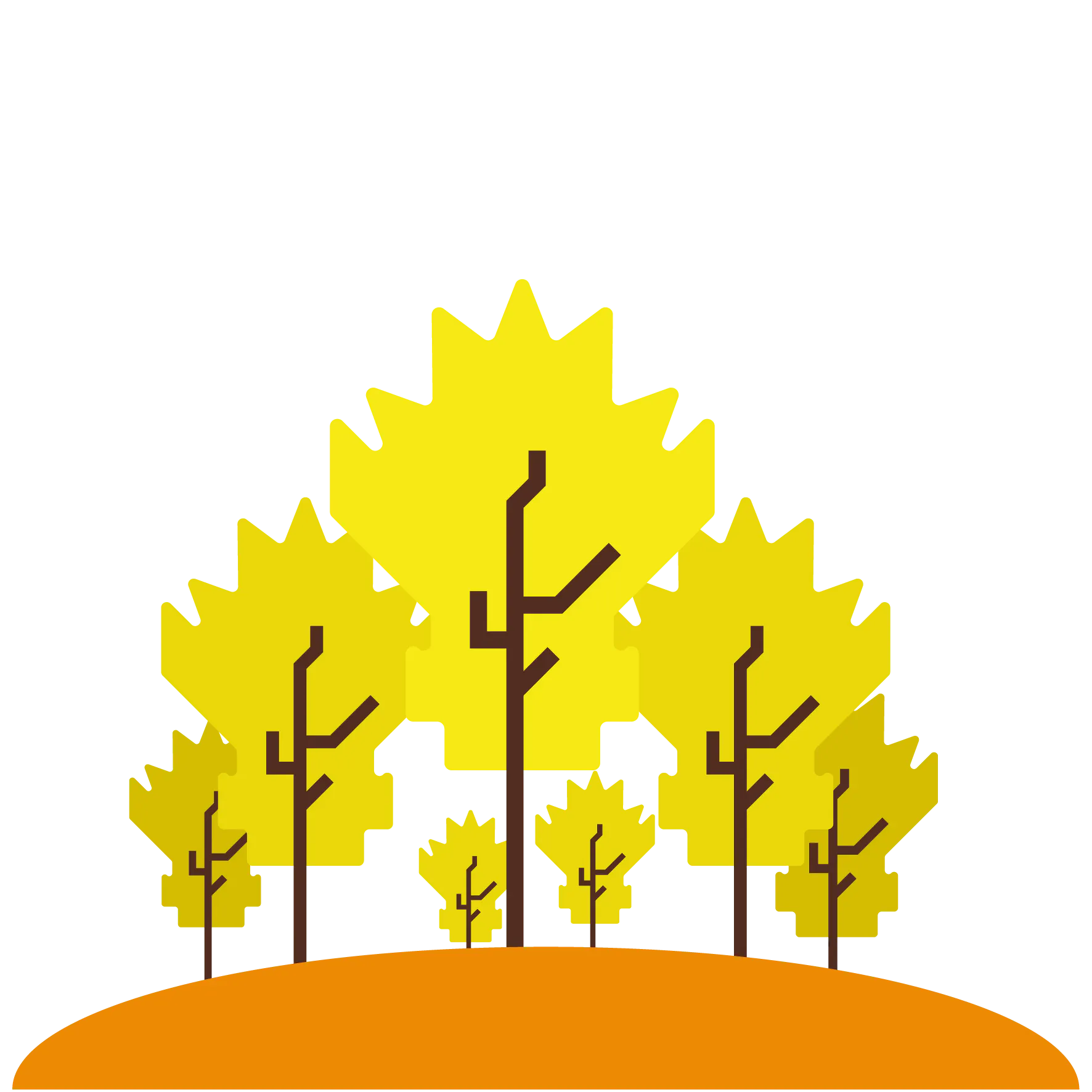# Arduino 101 Home BLE System

My project presents a domotic box with several features that can be controlled with an app, using Bluetooth low energy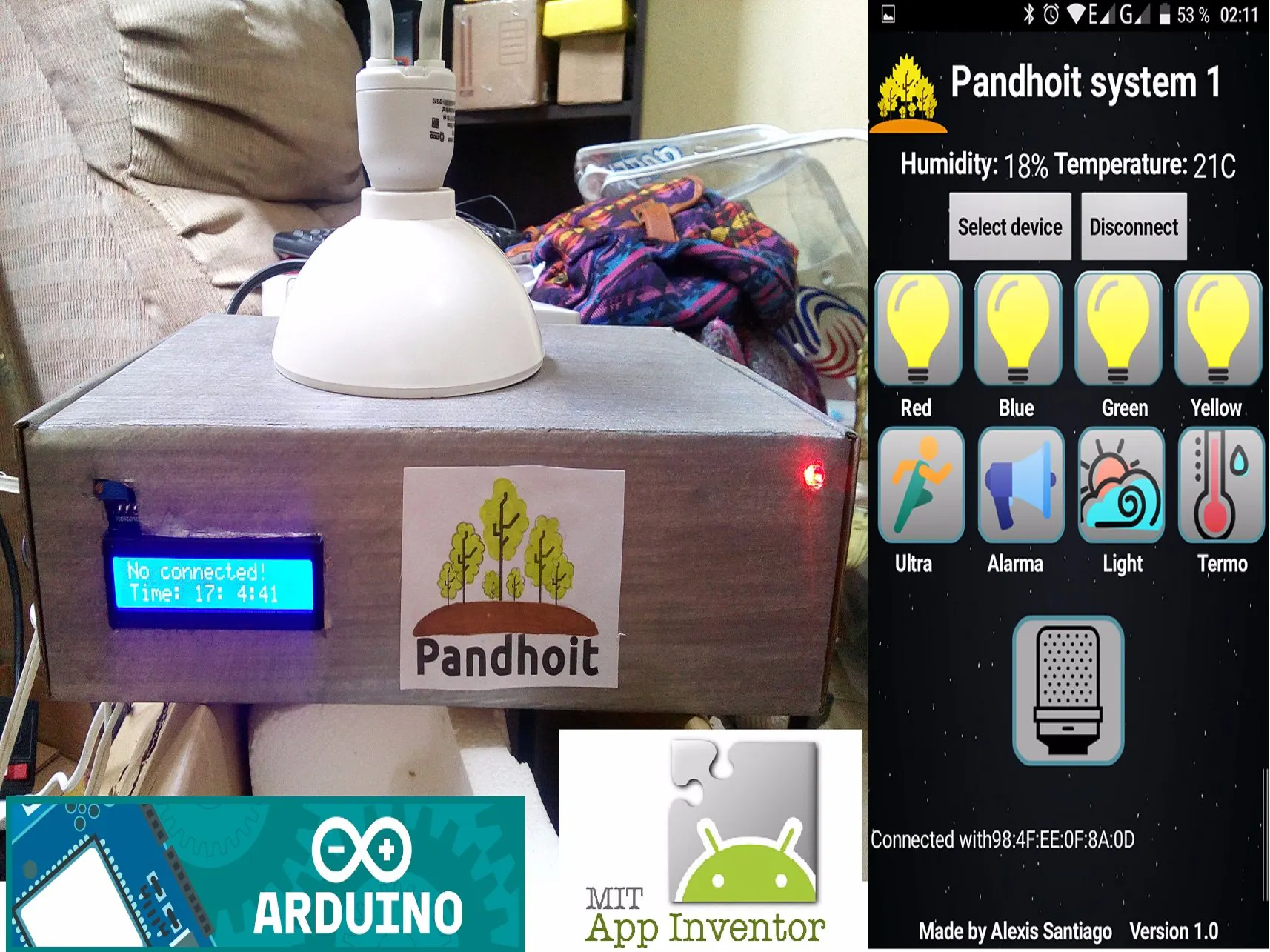## Things used in this project

### Hardware components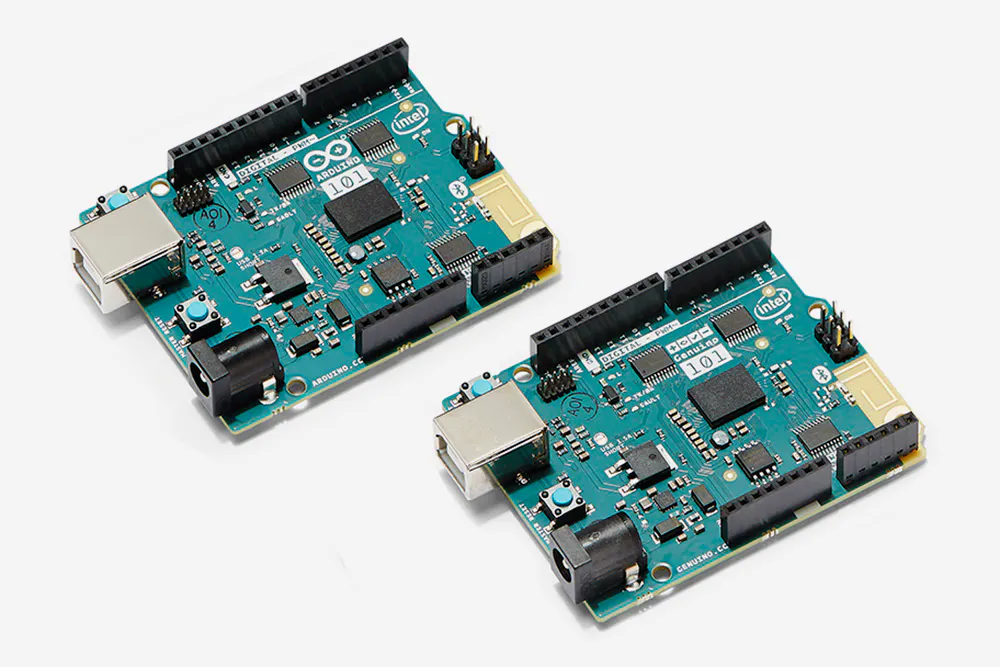Arduino 101 & Genuino 101
×1
 SmartThings relay 4 channel
×1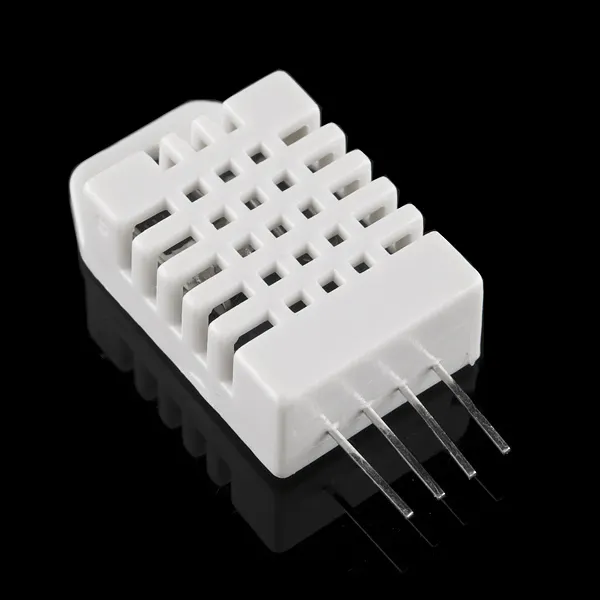DHT22 Temperature Sensor
×1Ultrasonic Sensor - HC-SR04 (Generic)
×1LED (generic)
×1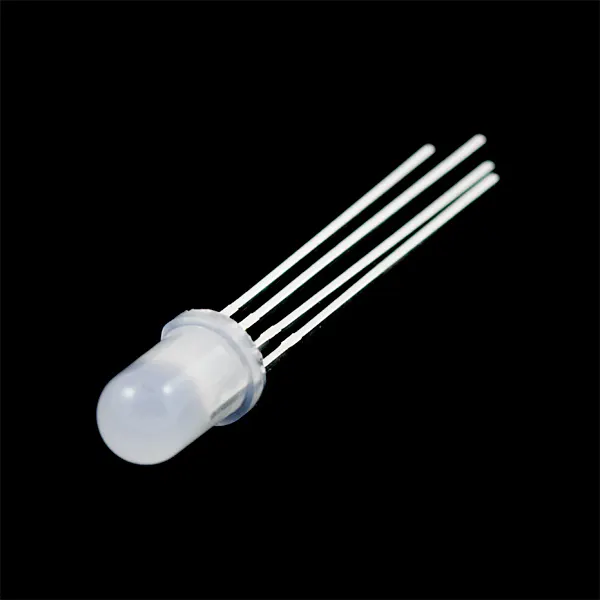RGB Diffused Common Cathode
×1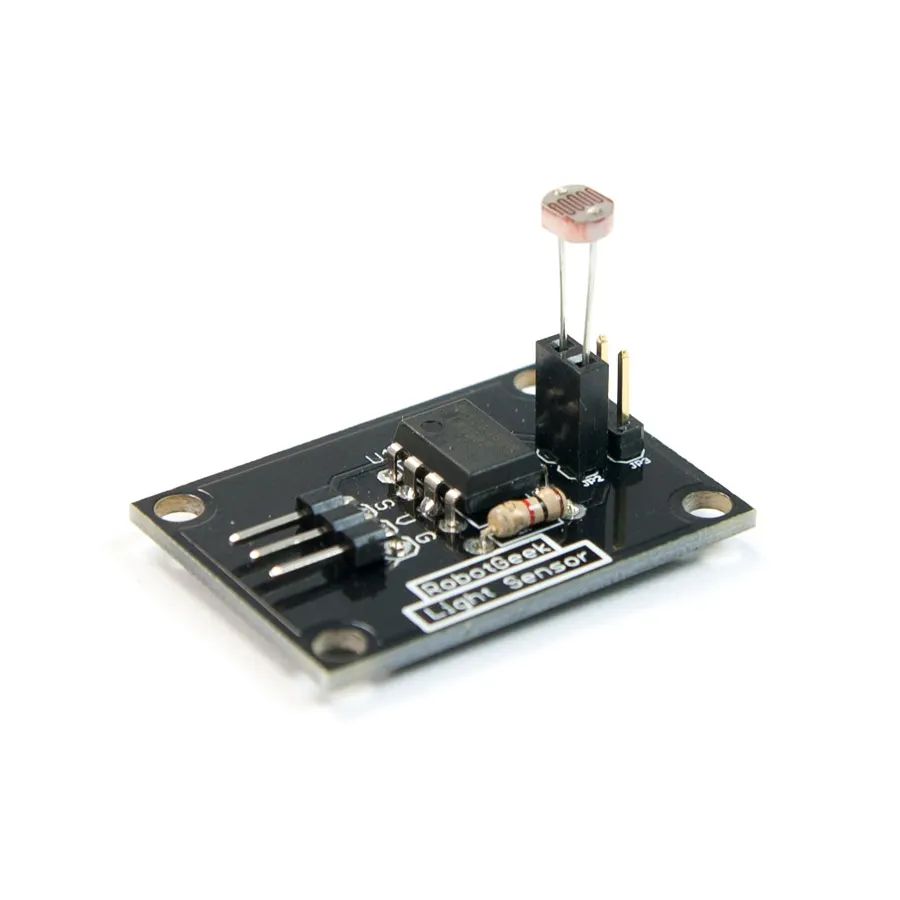RobotGeek Light Sensor
×1Resistor 10k ohm
×1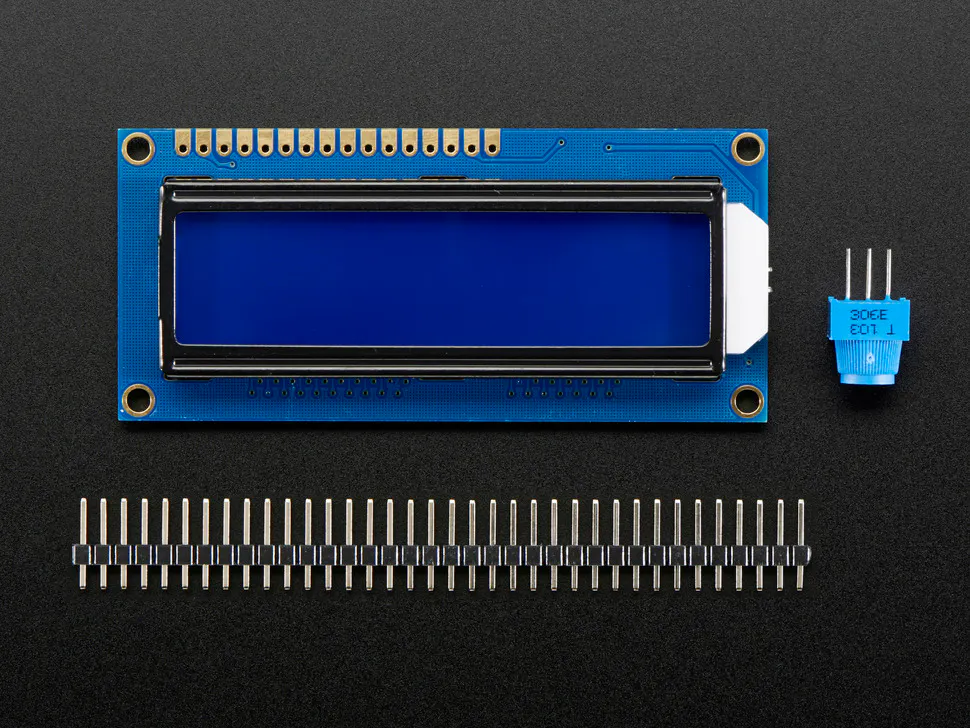Adafruit Standard LCD - 16x2 White on Blue
×1×1Jumper wires (generic)
×1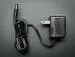9V 1A Switching Wall Power Supply
×1

### Software apps and online servicesArduino IDE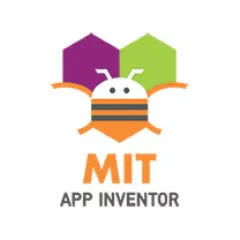MIT App Inventor 2

## Schematics

### Circuit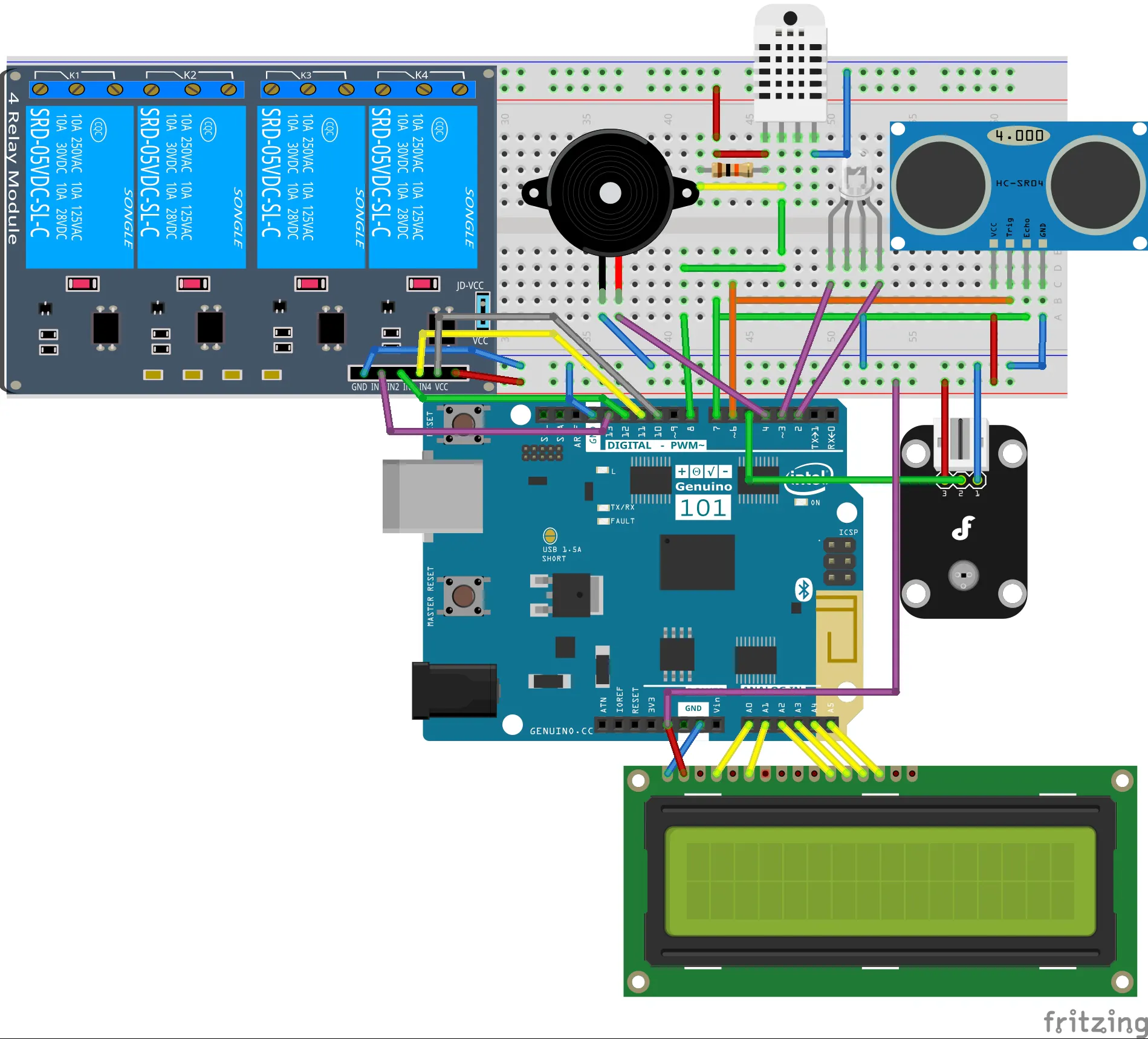## Code

### Pandhoit

Arduino
```/*Made by Alexis Santiago Allende Last Update 25/03/17 Pandhoit system version 1.0*/
#include <CurieBLE.h>
BLEPeripheral blePeripheral;//BLE Peripheral Device (Arduino Device)
BLEService pandhoit("19b10010-e8f2-537e-4f6c-d104768a1214"); // BLE pandhoit Service
//include the CurieTime Library
#include <CurieTime.h>
// BLE pandhoit buttons Characteristic - custom 128-bit UUID, read and writable by central
// BLE sensor rate Characteristic"
//DHT 22 library
#include "DHT.h"
#define DHTPIN 8
#define DHTTYPE DHT22
DHT dht(DHTPIN, DHTTYPE);
//LCD library
#include <LiquidCrystal.h>
LiquidCrystal lcd(15, 14, 19, 18, 17, 16);

long ts;// pin to use for time of ultasensor
long ds;// pin to use for distance
long previousMillis = 0;  // last time the sensor was checked, in ms
long previoustime = 0;  // last time the sensor was checked, in ms
const int c1 = 2; // pin to use for the light state
const int c2 = 3;// pin to use for the light state
const int c3 = 4; // pin to use for buzzer (alarm)
const int c4 = 5;// pin to use for light sensor
const int d1 = 13; // pin to use for the out control
const int d2 = 12;// pin to use for  the out control
const int d3 = 11; // pin to use for the out control
const int d4 = 10;// pin to use for the out control
const int d5 = 9; // pin to use for the out control (light sensor)
int send_data, datachoice; //  reading from sensor
//boolean variables for use to (a)button 1,(b)button 2,(c)button 3,(d)button 4,(e,g)button 5
//(f,g)button 7, (g) lighsensor,
boolean a = LOW, b = LOW,c=LOW,d=LOW,e=LOW,f=LOW,g=LOW,h=LOW,i=LOW,j=LOW,k=LOW,l=LOW,m=LOW,n=LOW,o=LOW,p=LOW,q=LOW; //Control variables
const int Trigger = 6;   //Pin digital 2 para el Trigger del sensor
const int Echo = 7;   //Pin digital 3 para el Echo del sensor

void setup() {
lcd.begin(16, 2);  // LCD starts
//set cursor to column 0, row 0
lcd.setCursor(0,0);
dht.begin();//sensor begin
//set time to 1:35:24 on April 4th, 2016. Can you change to your time / date
setTime(16, 56, 24, 3, 07, 2017);
pinMode(c1, OUTPUT);//light state
pinMode(c2, OUTPUT);//light state
pinMode(c3, OUTPUT);//alarm(buzzer)
pinMode(c4, INPUT); //light sensor
pinMode(d1, OUTPUT);//relay module output 1
pinMode(d2, OUTPUT);//relay module output 2
pinMode(d3, OUTPUT);//relay module output 3
pinMode(d4, OUTPUT);//relay module output 4 (light sensor control)
pinMode(d5, OUTPUT);
pinMode(Trigger, OUTPUT);
pinMode(Echo, INPUT);
digitalWrite(Trigger, LOW);
// set advertised local name and service UUID:
blePeripheral.setLocalName("Pandhoit");
// set the initial value for the characeristic:
buttons.setValue(1);
// begin advertising BLE Light service:
blePeripheral.begin();
digitalWrite(c1, HIGH);//Turn on the red indicator light
lcd.print("No connected!");     // print a simple message
//set cursor to column 0, row 1
lcd.setCursor(0,1);
lcd.print("Time:");     // print a message

}

void loop() {
//digitalWrite(c3, LOW);//alarm turn off
lightsens();//When the system is deactivated, the light sensor is activated for light control
ultra();//When the system is deactivated, the distance sensor is activated for intruder alarm control
//create a character array of 8 characters for the time
char clockTime;
//use sprintf to create a time string of the hour, minte and seconds
sprintf(clockTime, "%2d:%2d:%2d", hour(), minute(), second());
//set cursor to column 6, row 1
lcd.setCursor(6, 1);
//print the time string over lcd
lcd.print(clockTime);
// listen for BLE peripherals to connect:
BLECentral central = blePeripheral.central();
// if a central is connected to peripheral:
if (central) {
digitalWrite(c1, LOW);//Turn off the red indicator light
digitalWrite(c2, HIGH);//Turn on the blue indicator light

// while the central is still connected to peripheral:
while (central.connected()) {
if(o==LOW){
lcd.clear();//clear lcd display
lcd.setCursor(0,0);
lcd.print("App connected!");     // print a message
lcd.setCursor(0,1);
lcd.print("Temp:");     // print a message
lcd.setCursor(7,1);
lcd.print("C");     // print a message
lcd.setCursor(9,1);
lcd.print("Hum:");     // print a message
lcd.setCursor(15,1);
lcd.print("%");     // print a message
o=HIGH;
}

// if the remote device wrote to the characteristic,
// use the value to control the leds:
if (buttons.written()) {
change();// If in the app, I press a button then something happens
}
if (g==HIGH) {//if botton of light sensor was pressed then this sensor is activate to control d4 output
lightsens();
}
if (i==HIGH) {//if botton of temp sensor was pressed then this sensor is activate to control c3 alarm
}
if (l==HIGH) {//if botton of ultrasonic sensor was pressed then this sensor is activate to control c3 alarm
ultra();
}

long currentMillis = millis();
// if 10s have passed, check the sensor:
if (currentMillis - previousMillis >= 10000) {
previousMillis = currentMillis;
}
//finish
}
// when the central disconnects, print it out:
Serial.print(F("Disconnected from central: "));
digitalWrite(c1, HIGH);//Turn on the red indicator light
digitalWrite(c2, LOW);////Turn off the blue indicator light
if(o==HIGH){
lcd.clear();
lcd.setCursor(0,0);
lcd.print("No connected!");     // print a message
lcd.setCursor(0,1);
lcd.print("Time:");     // print a message
o=LOW;
}
}
else
{
digitalWrite(c1, HIGH);//Turn on the red indicator light
digitalWrite(c2, LOW);////Turn off the blue indicator light

}
}

if (datachoice == 0)
{
send_data = tempe;//send temperature
datachoice = 1;
lcd.setCursor(5,1);
lcd.print(tempe);     // print a message

}
else if(datachoice == 1)
{
datachoice = 0;
lcd.setCursor(13,1);
}

sensors.setValue(send_data);

}

void lightsens()//function to able and disabled lightsensor
{
{
digitalWrite(d4, LOW);
}
else
{
digitalWrite(d4, HIGH);
}
}

void change()//function to read values obtained in the app
{
if (buttons.value() == '1' && a == LOW) { // 1 in ASCII

digitalWrite(d1, HIGH);         // will turn the LED on
a = HIGH;
} else if (buttons.value() == '1' && a == HIGH)  {  //when 1 was read again (second time)

digitalWrite(d1, LOW);          // will turn the LED off
a = LOW;
}

if (buttons.value() == '2' && b == LOW) { // 2 in ASCII

digitalWrite(d2, HIGH);         // will turn the LED on
b = HIGH;
} else if (buttons.value() == '2' && b == HIGH)  { //when 2 was read again (second time)

digitalWrite(d2, LOW);          // will turn the LED off
b = LOW;
}
if (buttons.value() == '3' && c == LOW) { // 3 in ASCII

digitalWrite(d3, HIGH);         // will turn the LED on
c = HIGH;
} else if (buttons.value() == '3' && c == HIGH)  { //when 3 was read again (second time)

digitalWrite(d3, LOW);          // will turn the LED off
c = LOW;
}

if (buttons.value() == '4' && e == LOW&& g==LOW) { // 4 in ASCII

digitalWrite(d4, HIGH);         // will turn the LED off
e = HIGH;
} else if (buttons.value() == '4' && e == HIGH && g==LOW)  { //when 4 was read again (second time)

digitalWrite(d4, LOW);          // will turn the LED off
e = LOW;
}

if (buttons.value() == '5' && d == LOW) { // 5 in ASCII

l = HIGH;//able ultrasonic sensor
d=HIGH;
} else if (buttons.value() == '5'&& d==HIGH)  { //when 5 was read again (second time)

l = LOW;//disable ultrasonic sensor
d=LOW;
}
if (buttons.value() == '6' && h == LOW && i==LOW) { // 6 in ASCII

digitalWrite(c3, HIGH);         // will turn the alarm(buzzer) on
h = HIGH;
} else if (buttons.value() == '6' && h == HIGH && i==LOW)  { //when 6 was read again (second time)

digitalWrite(c3, LOW);          // will turn the alarm(buzzer) off
h = LOW;
}
if (buttons.value() == '7' && f == LOW) { // 7 in ASCII

g = HIGH;//able light sensor
f=HIGH;
} else if (buttons.value() == '7'&&f==HIGH)  { //when 7 was read again (second time)

g = LOW;//disable light sensor
f=LOW;
}
if (buttons.value() == '8' && j == LOW) { // 8 in ASCII

i = HIGH;//able temperature sensor
j=HIGH;
} else if (buttons.value() == '8'&& j==HIGH)  { //when 8 was read again (second time)

i = LOW;//disable temperature sensor
j=LOW;
}

}
void ultra(){//function to read and control ultrasonic sensor
digitalWrite(Trigger, HIGH);
delayMicroseconds(10);          //Send a pulse of 10us
digitalWrite(Trigger, LOW);

ts = pulseIn(Echo, HIGH); //obtain width of pulse
ds = ts/59;             //change value to centimeters
if(ds<30
&& m==LOW){//if distance is lower than 5 centimeter then able  alarm
digitalWrite(c3,HIGH);
m=HIGH;
n=LOW;
}
else if(ds>=30&&n==LOW)//if distance is upper than 5 centimeter then disable  alarm
{
digitalWrite(c3,LOW);
n=HIGH;
m=LOW;
}
}

void tempalert()//function to control temperature between 24 and 25 degrees
{
if(tempe>=25 && p==LOW)//if temperature above of 25 degrees
{
digitalWrite(c3,HIGH);//turn on alarm
p=HIGH;
q=LOW;

}
else if(tempe<=24&&q==LOW)//if temperature is under 24 degrees
{
p=LOW;
q=HIGH;
digitalWrite(c3,LOW);//turn off alarm
}

}
```

### Pandhoit.aia

Arduino
App inventor project code
`No preview (download only).`

## Credits

### Alexis Santiago Allende

0 projects • 60 followers
Im a person who since young feel a passion for electronics, I also like to cook pizza and travel. Now Im working on the internet of things